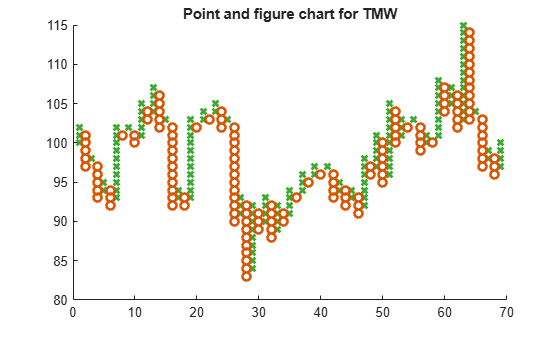Main Content

# pointfig

Point and figure chart

`pointfig` is updated to accept data input as a matrix, `timetable`, or `table`.

## Syntax

``pointfig(Data)``
``h = pointfig(ax,Data)``

## Description

example

````pointfig(Data)` plots a point and figure chart from a series of prices of a security. Upward price movements are plotted as `X`'s and downward price movements are plotted as `O`'s.```

example

````h = pointfig(ax,Data)` adds an optional argument for `ax`. ```

## Examples

collapse all

Load the file `SimulatedStock.mat`, which provides a timetable (`TMW`) for financial data for TMW stock. This Point and Figure chart is for closing prices of the stock TMW for the most recent 21 days. Note that the variable name of asset price is be renamed to `'Price'` (case insensitive).

```load SimulatedStock.mat TMW.Properties.VariableNames{'Close'} = 'Price'; pointfig(TMW(1:200,:)) title('Point and figure chart for TMW')```## Input Arguments

collapse all

Data for a series of prices, specified as a matrix, table, or timetable. Timetables and tables with `M` rows must contain a variable named `'Price'` (case insensitive).

Data Types: `double` | `table` | `timetable`

(Optional) Valid axis object, specified as an axes object. The point and figure plot is created in the axes specified by `ax` instead of in the current axes (`ax = gca`). The option `ax` can precede any of the input argument combinations.

Data Types: `object`

## Output Arguments

collapse all

Graphic handle of the figure, returned as a handle object.

## See Also

### Topics

Introduced in R2006a

Download ebook